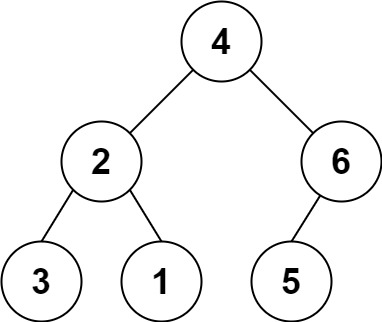536. Construct Binary Tree from String

You need to construct a binary tree from a string consisting of parenthesis and integers.

The whole input represents a binary tree. It contains an integer followed by zero, one or two pairs of parenthesis. The integer represents the root's value and a pair of parenthesis contains a child binary tree with the same structure.

You always start to construct the left child node of the parent first if it exists.

Example 1:```Input: s = "4(2(3)(1))(6(5))"
Output: [4,2,6,3,1,5]
```

Example 2:

```Input: s = "4(2(3)(1))(6(5)(7))"
Output: [4,2,6,3,1,5,7]
```

Example 3:

```Input: s = "-4(2(3)(1))(6(5)(7))"
Output: [-4,2,6,3,1,5,7]
```

Constraints:

• `0 <= s.length <= 3 * 104`
• `s` consists of digits, `'('`, `')'`, and `'-'` only.

536. Construct Binary Tree from String
``````struct Solution;
use rustgym_util::*;
use std::iter::Peekable;
use std::vec::IntoIter;

enum Tok {
Num(i32),
Op(char),
}

use Tok::*;

struct TreeParser {
it: Peekable<IntoIter<Tok>>,
}

impl TreeParser {
fn new(s: String) -> Self {
let mut tokens = vec![];
let mut it = s.chars().peekable();
while let Some(c) = it.next() {
match c {
'0'..='9' => {
let mut val = (c as u8 - b'0') as i32;
while let Some(&c) = it.peek() {
match c {
'0'..='9' => {
it.next();
val *= 10;
val += (c as u8 - b'0') as i32;
}
_ => {
break;
}
}
}
tokens.push(Num(val));
}
_ => {
tokens.push(Op(c));
}
}
}
let it = tokens.into_iter().peekable();
TreeParser { it }
}

fn parse(&mut self) -> TreeLink {
let sign = if self.eat('-') { -1 } else { 1 };
if let Some(Num(val)) = self.it.next() {
let mut left = None;
let mut right = None;
if self.eat('(') {
left = self.parse();
self.eat(')');
}
if self.eat('(') {
right = self.parse();
self.eat(')');
}
tree!(val * sign, left, right)
} else {
None
}
}

fn eat(&mut self, c: char) -> bool {
if let Some(&Op(t)) = self.it.peek() {
if t == c {
self.it.next();
return true;
}
}
false
}
}

impl Solution {
fn str2tree(s: String) -> TreeLink {
TreeParser::new(s).parse()
}
}

#[test]
fn test() {
let s = "4(2(3)(1))(6(5))".to_string();
let res = tree!(4, tree!(2, tree!(3), tree!(1)), tree!(6, tree!(5), None));
assert_eq!(Solution::str2tree(s), res);
let s = "-4(2(3)(1))(6(5))".to_string();
let res = tree!(-4, tree!(2, tree!(3), tree!(1)), tree!(6, tree!(5), None));
assert_eq!(Solution::str2tree(s), res);
}
``````